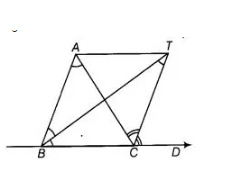# Bisectors of interior ∠B and exterior`
Question:

Bisectors of interior ∠B and exterior ∠ACD of a  ΔABC intersect at the point T. Prove that ∠BTC = ½ ∠BAC.

Thinking Process

For obtaining the interior required result use the property that the exterior angle of a triangle is equal to the sum of the two opposite angles of triangle.Solution:

Given In AABC, produce SC to D and the bisectors of  ∠ABC and ∠ACD meet at point T. To prove  ∠BTC = ½ ∠BAC

Proof $\ln \triangle A B C, \angle C$ is an exterior angle.

$\therefore$ $\angle A C D=\angle A B C+\angle C A B$

[exterior angle of a triangle is equal to the sum of two opposite angles]

$\Rightarrow$ $\frac{1}{2} \angle A C D=\frac{1}{2} \angle C A B+\frac{1}{2} \angle A B C$ [dividing both sides by 2]

$\Rightarrow$ $\angle T C D=\frac{1}{2} \angle C A B+\frac{1}{2} \angle A B C$ $\ldots(1)$

$\left[\because C T\right.$ is a bisector of $\left.\angle A C D \Rightarrow \frac{1}{2} \angle A C D=\angle T C D\right]$

In $\triangle B T C, \quad \angle T C D=\angle B T C+\angle C B T$

[exterior angle of a triangle is equal to the sum of two opposite interior angles]

$\Rightarrow$ $\angle T C D=\angle B T C+\frac{1}{2} \angle A B C$ ...(ii)

$\left[\because B T\right.$ bisects of $\left.\angle A B C \Rightarrow \angle C B T=\frac{1}{2} \angle A B C\right]$

From Eqs. (i) and (ii),

$\frac{1}{2} \angle C A B+\frac{1}{2} \angle A B C=\angle B T C+\frac{1}{2} \angle A B C$

$\Rightarrow \quad \angle B T C=\frac{1}{2} \angle C A B$

or $\angle B T C=\frac{1}{2} \angle B A C$